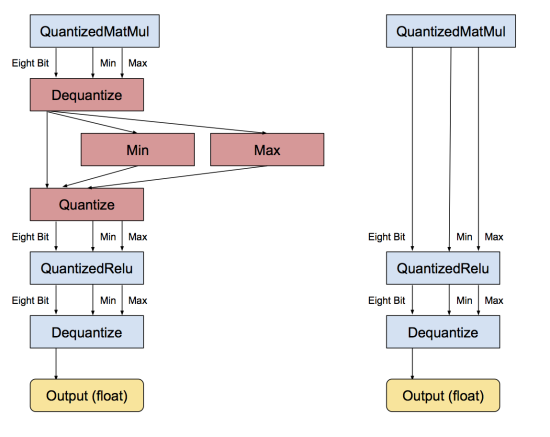# 【论文阅读笔记】Quantization and Training of Neural Networks for Efficient Integer-Arithmetic-Only Inference

该论文提出了一种允许网络在推测时只使用整数计算的方法，即 float32 --> int8.
该文在MobileNets等已经压缩过的网络进行量化，测试数据集为ImageNet分类数据集。不同于其他的方法，在高度冗余的模型，如Alexnet等网络上进行的，且提出的硬件加速方法需要专门的特殊硬件（即他们并没有在真正的硬件上加速，只是提出一种理论），该方法在普通的CPU上即可运行。

Introduction

以前的方法在两方面减少modle size和推测时间：

1. 网络结构上，如MobileNet、SqueezeNet、ShuffleNet和DenseNet
2. 量化权重和激活函数，从32 bit的floating point到更低的 bit-depth表示

但是之前的方法有两个方面的缺陷：

2. **很多量化放大没有真正在硬件上进行有效性证明。**① 有的方法只在weight上量化，仅仅在乎在设备的存储，而不在乎计算效率；② 有的方法，比如二元/三元权重网络和bit-shift网络，他们的方法把权重限制为0或者2n，他们想把乘法计算用bit-shift实现。但是，在现有的硬件上，bit-shift实现并不比<乘法-加法>好。而且，只有当bit较大时，乘法才显得“昂贵”，所以，我们需要将乘法的输入—weight和activation都给量化成较小的bit-width。

Quantized Inference

Quantized scheme

作者的量化方案为：在测试时只使用int计算，在训练时使用浮点计算。
量化方案的基本要求就是：允许只用量化值的整数运算来完成所有的算术运算。
量化方案是一个仿射映射，在整数 q q 和实值 r r r = S ( q − Z ) (1) r = S(q-Z) \tag{1}   其中 S 和 Z S和Z 是常数 S 和 Z S和Z 是量化参数）【对每一层array用一对S/Z参数，即每一个activation、每一个weight的Tensor都有一个对应S/Z】

对于8-bit的量化，q就是8-bit的整数。对于B-bit的量化，q就是B-bit的实数。
而对于bias，就固定量化为32-bit的实数。

常数 S S step_size，代表一个“scale”，是一个实数。常数 Z Z 是偏移shift，代表“zero-point”，就是为了让实数 r r 0 0 和量化数 q q 0 0 对应，和q是一个类型的数。 S = a r r a y m a x − a r r a y m i n 2 b i t _ w i d t h − 1 (9) S=\frac{array_{max}-array_{min}}{2^{bit\_width}-1}\tag{9} 设定： r o u n d ( x ) = { 0 , x < 0 ⌊ x ⌉ ， 0 < ⌊ x ⌉ < 2 n − 1 2 n − 1 , x > 2 n − 1 round(x) = \begin{cases}0,&x<0 \\ \lfloor x \rceil， &0<\lfloor x \rceil<2^{n}-1 \\ 2^{n}-1, &x>2^{n}-1\end{cases}
Z = r o u n d ( − a r r a y m i n S ) (10) Z=round(-\frac{array_{min}}{S})\tag{10}

template<typename QType>                    // e.q.  QType=uint8
struch QuantizedBuffer{
vector<QType> q;                        // the quantized values
float S;                                // the scale
QType Z;                                // the zero-point
}


1. 输入 量化的特征图 lhs_quantized_val, uint8类型, 偏移量 lhs_zero_point, int32类型;
2. 输入 量化的卷积核 rhs_quantized_val, uint8类型, 偏移量 rhs_zero_point, int32类型;
3. 转换 unit8 到 int32类型;
4. 每一块卷积求和(int32乘法求和有溢出风险，可换成固定点小数树乘法);
int32_accumulator += (lhs_quantized_val(i, j) - lhs_zero_point) * (rhs_quantized_val(j, k) - rhs_zero_point);
5. 输入 量化的乘子 quantized_multiplier, int32类型 和 右移次数记录 right_shift, int类型;
6. 计算乘法，得到int32类型的结果 (int32乘法有溢出风险，可换成固定点小数树乘法);
quantized_multiplier * int32_accumulator

7. 再左移动 right_shift 位还原，得到 int32的结果;
8. 最后再加上 结果的偏移量 result_zero_point;
(7和8的顺序和 官方说的先后顺序颠倒);
9. 将int32类型结果 限幅到[0, 255], 再强制转换到 uint8类型;
10. 之后再 反量化到浮点数，更新统计输出值分布信息 max, min;
11. 再量化回 uint8;
11. 之后 经过 量化激活层;
12. 最后 反量化到 浮点数，本层网络输出;

13. 进入下一层
循环执行 1~12 步骤


若有连续的层需要量化操作时，就没必要进行反量化了。。比如上面步骤的 10 → 11 10\rightarrow11 ，实际上是可以省略的【但相应的。。可能会带来乘法加法累积造成的溢出】Integer-arithmetic-only matrix multiplication

由公式 ( 1 ) (1) 可以知道，每个array中的实数 r i r_i 都能表示带有一对参数 S 和 Z S和Z 的实数 q i q_i 。则对实数矩阵 R 1 和 R 2 R_1和R2 的乘法，其结果矩阵的每个实数可以表示为： S 3 ( q 3 ( i , k ) − Z 3 ) = ∑ j = 1 N S 1 ( q 1 ( i , j ) − Z 1 ) S 2 ( q 2 ( j , k ) − Z 2 ) (2) S_3(q_3^{(i,k)}-Z_3)=\sum_{j=1}^{N}S_1(q_1^{(i,j)}-Z_1)S_2(q_2^{(j,k)}-Z_2)\tag{2} q 3 ( i , k ) = Z 3 + M ∑ j = 1 N ( q 1 ( i , j ) − Z 1 ) ( q 2 ( j , k ) − Z 2 ) (3) q_3^{(i,k)}=Z_3+M\sum_{j=1}^{N}(q_1^{(i,j)}-Z_1)(q_2^{(j,k)}-Z_2)\tag{3} 其中， M = S 1 S 2 S 3 (4) M=\frac{S_1S_2}{S_3}\tag{4} M M 是公式 ( 3 ) (3) 中唯一不是整数的值。经验发现， M M 总是在 ( 0 , 1 ) (0,1) 中，所以将 M M 表达为： M = 2 − n M 0 (5) M=2^{-n}M_0\tag{5}   其中， n n 为非负整数， M 0 M_0 是一个整数。这样，实数运算就变成了整数运算。同时， 2 − n 可 以 2^{-n}可以 用移位运算。因为图片输入的每一个数字都在 [ 0 , 255 ] [0,255] ，而映射的区域也是 [ 0 , 2 8 − 1 ] = [ 0 , 255 ] [0, 2^8-1]=[0,255]

Implementation of a typical fused layer

定义bias-vector的量化： S b i a s = S 1 S 2 ， Z b i a s = 0 S_{bias}=S_1S_2，Z_{bias}=0 其中， S b i a s S_{bias} 用int32表示。
矩阵乘法，weights和activations的矩阵乘法后的加法： i n t 32 + = u i n t 8 ∗ u i n t 8 int32 += uint8 *uint8
使用32位加法器得结构后，还有3件事情需要做：① 将这个output activations的int32 scale downint8scale指标；② 将 int32 的output activations 仿射到 uint8；③ 对这个uint8的结果应用激活函数。
这个乘法的down-scaling就是公式 ( 3 ) 的 (3)的 M M .

Training with simulated quantization

Quantized scheme

作者分析原有的量化方法：用floating point训练，然后量化训练的weight.(有时候会进行量化后的微调)，发现这种方法对于大模型来说结果不错，对小模型来说精确度下降不小。作者对这种量化后的微调方法分析了存在的两点问题：

1. 同一layers不同channels权重分布尺度差很多（100x）
1. 离散的权重会导致所有剩余的权重的精度下降；

作者提出了一种在前向传播阶段模拟量化的方法，反向传播和平常一样，所有的权重和biases都用floating point保存以微调小变化。前向传播的浮点数计算方法，模拟量化的方式，就如公式 ( 3 ) (3) 所示

对每一层，数值都用参数（量化层次，和clamping range）量化： c l a m p ( r ; a , b ) : = m i n ( m a x ( x , a ) , b ) (6) clamp(r;a,b) := min(max(x,a),b)\tag{6} s ( a , b , n ) : = b − a n − 1 (7) s(a,b,n):=\frac{b-a}{n-1} \tag{7} q ( r ; a , b , n ) : = ⌊ c l a m p ( r ; a , b ) − a s ( a , b , n ) ⌉ s ( a , b , n ) + a (8) q(r;a,b,n):=\lfloor\frac{clamp(r;a,b)-a}{s(a,b,n)}\rceil s(a,b,n)+a\tag{8} 其中， r r 是待量化的实数； [ a , b ] [a,b] 是量化的范围； n n 是量化的等级数，对所有layer都是固定的，比如8-bit量化， n = 2 8 = 256 n=2^8=256 ⌊ ∗ ⌉ \lfloor * \rceil 就是取整到最近的整数。
公式 ( 6 ) (6) 表示x所处的范围，即映射前的range，之所以有这个看起来没用的公式，是因为activation的range是移动均值平均的出来的；公式 ( 7 ) (7) 表示range映射到n-1段，每段的大小；公式 ( 8 ) (8) 表示一个实数r就近到能完整量化的实数，且 ⌊ c l a m p ( r ; a , b ) − a s ( a , b , n ) ⌉ \lfloor\frac{clamp(r;a,b)-a}{s(a,b,n)}\rceil 是能我们的B-bit表示的量化结果；

bias没有被量化，因为在推测阶段，它被32-bit表示。【这句没懂，因为前面不是有量化bias的参数 S b i a s S_{bias} 吗？】

Learning quantization ranges
weight 和 activation的量化range不同：

• 对于weight： a : = m i n ( w ) a:=min(w) b : = m a x ( w ) b:=max(w)
• 对于activation：在训练时，用指数移动平均来收集activation的range [a,b]，平滑参数接近1.【若范围快速变化时，指数移动平均会延迟反映出来，所以在训练初期（5万步到200万步）时，不量化activation是有用的】
11-1153
02-26643
01-194299
12-251170
11-20794
03-22781
09-208998
03-31686
04-29193
12-16444
11-091350
01-171081
12-226110
04-03262
02-06933
09-053683
04-07790
09-24863
06-182445
03-281万+

### “相关推荐”对你有帮助么？

•非常没帮助
•没帮助
•一般
•有帮助
•非常有帮助被折叠的  条评论 为什么被折叠?到【灌水乐园】发言¥2 ¥4 ¥6 ¥10 ¥20余额支付 (余额：-- )扫码支付获取中扫码支付点击重新获取扫码支付1.余额是钱包充值的虚拟货币，按照1:1的比例进行支付金额的抵扣。
2.余额无法直接购买下载，可以购买VIP、C币套餐、付费专栏及课程。余额充值